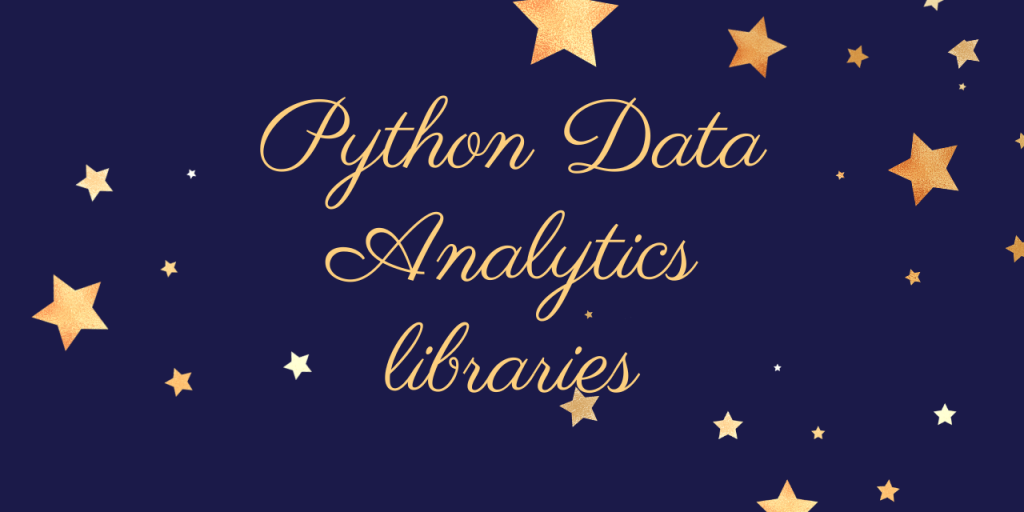# 4 Python Data Analytics libraries to know!Hello, readers! In this article, we will be focusing on 4 Python Data Analytics libraries, in detail.

So, let us get started!! 🙂

## Data Analytics – Quick Overview!

Prior to understanding Python libraries that support the functioning of Data Analytics, it is very important to understand the concept of Data Analytics.

Data Analysis is altogether a sub-domain under the primary domain of Data Science and Machine Learning. Yes, prior to model the data against various algorithms, it is very important to analyze the data and clean the data.

With analyzing the data, we mean to say that the data needs to be understood in terms of the distribution, statistical analysis of measurement and also the visualization of the data for a clear picture of the data.

Analysis of data includes,

• Cleaning the data
• Understanding the distribution of the data values
• Statistical analysis of the data against mean, standard deviation, etc.
• Visualization of the data values against the statistical measures.
• Formatting the data for processing into the model.

## Python Data Analytics libraries

With Python comes a huge list of libraries that supports the concept of data Analytics. Yes, Python offers a huge range of modules to carry out the pre-processing and analysis of data values.

In context to the current topic, we will be covering the below mostly used Python libraries to perform data analytics related tasks–

### 1. Scikit-learn

Python Scikit-learn library, open source library, is the choice of most of the data science or machine learning engineers for data analysis. This library provides wide range of functions to perform data pre-processing as well analysis efficiently.

It is actually constructed over the NumPy, Matplotlib and SciPy libraries of Python. With Scikit-learn library, comes a list of algorithms to perform statistical modelling also other machine learning related algorithms such as —

1. Regression models
2. Statistical data processing
3. Preprocessing functions
4. Clustering models
5. Classification models, etc.

It also includes various supervised ML as well Unsupervised ML algorithms.

### 2. OpenCV

Python OpenCV ( Open Source Computer Vision) is an extensively used algorithm for data analytics. With OpenCV, our data analytics does not remain confined to structured data. That is, with OpenCV in place, we can perform analysis of images, pictures, and videos too.

Thus OpenCV supports the following–

• Facial recognition
• object identification
• tracking the motion & mobility, etc.

We can make use of OpenCV to extract meaningful information from the data to be analyzed, also enables us to have predictive analysis on the data values.

### 3. Pandas

Python Pandas module offers us different functions to perform data analysis using Python. It is an acronym for Python Data Analysis Library.

With Pandas, we can easily perform the pre-processing of the data as well as analyze it against various parameters such as,

• Outliers
• Missing value analysis, etc.

It is based over the NumPy library that gives us an upper hand for mathematical operations too. Pandas library makes use of a data structure named DataFrame that basically gets the data into a tabular format and we can analyze the data in the form of rows and columns.

### 4. PyBrain

PyBrain is an acronym for Python Based Reinforcement Learning, Artificial Intelligence and Neural network library. The beauty of PyBrain is that it supports pre-defined environments to perform analysis and define relation between algorithms between models.

It supports various data analysis algorithms to enhance the analysis of the data and also test the outcome based on various scenarios.

## Conclusion

By this, we have come to the end of this topic. Feel free to comment below, in case you come across any question.

For more such posts related to Python programming, Stay tuned with us.

Till then, Happy Learning!! 🙂